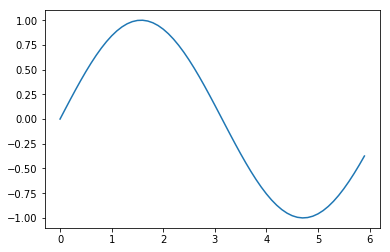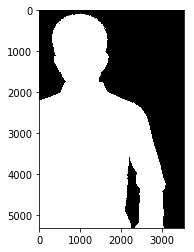# 深度学习之《深度学习入门》学习笔记（一）Python入门

2019/08/12 21:41 下午 posted in  随记

## NumPy

import sys, os
sys.path.append('../input/deeplearningfromscratch/deeplearningfromscratch')  # 为了导入父目录中的文件而进行的设定

import numpy as np
x= np.array([1.0, 2.0, 3.0])
print(x)


[1. 2. 3.]

type(x)


numpy.ndarray

# 下面是NumPy数组的算术运算例子
x = np.array([1.0, 2.0, 3.0])
y = np.array([2.0, 3.0, 4.0])
print(x + y)
print(x - y)
print(x * y) # element-wise porduct
print(x / y)


[3. 5. 7.]
[-1. -1. -1.]
[ 2. 6. 12.]
[0.5 0.66666667 0.75 ]

NumPy数组也可以和单一的数值（标量）组合起来进行运算。此时，需要在NumPy数组的各个元素和标量之间进行运算。这个功能也被称为广播

x = np.array([1.0, 2.0, 3.0])
print(x / 2.0)


[0.5 1. 1.5]

# NumPy的多维数组
A = np.array([[1, 2],[3, 4]])
print(A)


[[1 2]
[3 4]]

A.shape


(2, 2)

A.dtype


dtype('int64')

# 矩阵的算术运算
B = np.array([[3, 0],[0,6]])
A+B


array([[ 4, 2],
[ 3, 10]])

A*B


array([[ 3, 0],
[ 0, 24]])

print(A)
A*10


[[1 2]
[3 4]]

array([[10, 20],
[30, 40]])

# 一个广播的例子
A = np.array([[1, 2], [3, 4]])
B = np.array([10, 20])
A * B


array([[10, 40],
[30, 80]])

# 访问元素
X = np.array([[51, 55],[14,19],[0,4]])
print(X)


[[51 55]
[14 19]
[ 0 4]]

for row in X:
print(row)


[51 55]
[14 19]
[0 4]

# 使用数组访问各个元素
X = X.flatten() # 将X转换为一维数组
print(X)


[51 55 14 19 0 4]

X[np.array([0,2,4])] #获取索引为0、2、4的元素


array([51, 14, 0])

# 从X中抽取大于15的元素
X > 15


array([ True, True, False, True, False, False])

X[X>15]


array([51, 55, 19])

## Matplotlib

import numpy as np
import matplotlib.pyplot as plt

#生成数据
x = np.arange(0, 6, 0.1) #生成0-6的数据，步长为0.1
y = np.sin(x)
#绘制图像
plt.plot(x,y)
plt.show()# 尝试追加cos函数的图形，并尝试使用pyplot的添加标题和x轴标签名等其他功能
y2 = np.cos(x)

plt.plot(x, y, label="sin")
plt.plot(x, y2, linestyle = "--", label="cos")#用虚线绘制
plt.xlabel("x")
plt.ylabel("Y")
plt.title('sin & cos')
plt.legend()
plt.show()pyplot中还提供了用于显示图像的方法imshow()。另外，可以使用matplotlib.image模块的imread()方法读入图像。

from matplotlib.image import imread